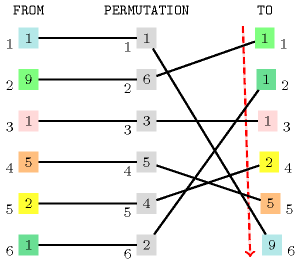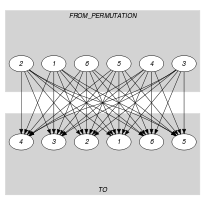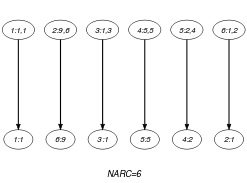## 5.372. sort_permutation

Origin
Constraint

$\mathrm{𝚜𝚘𝚛𝚝}_\mathrm{𝚙𝚎𝚛𝚖𝚞𝚝𝚊𝚝𝚒𝚘𝚗}\left(\mathrm{𝙵𝚁𝙾𝙼},\mathrm{𝙿𝙴𝚁𝙼𝚄𝚃𝙰𝚃𝙸𝙾𝙽},\mathrm{𝚃𝙾}\right)$

Usual name

$\mathrm{𝚜𝚘𝚛𝚝}$

Synonyms

$\mathrm{𝚎𝚡𝚝𝚎𝚗𝚍𝚎𝚍}_\mathrm{𝚜𝚘𝚛𝚝𝚎𝚍𝚗𝚎𝚜𝚜}$, $\mathrm{𝚜𝚘𝚛𝚝𝚎𝚍𝚗𝚎𝚜𝚜}$, $\mathrm{𝚜𝚘𝚛𝚝𝚎𝚍}$, $\mathrm{𝚜𝚘𝚛𝚝𝚒𝚗𝚐}$.

Arguments
 $\mathrm{𝙵𝚁𝙾𝙼}$ $\mathrm{𝚌𝚘𝚕𝚕𝚎𝚌𝚝𝚒𝚘𝚗}\left(\mathrm{𝚟𝚊𝚛}-\mathrm{𝚍𝚟𝚊𝚛}\right)$ $\mathrm{𝙿𝙴𝚁𝙼𝚄𝚃𝙰𝚃𝙸𝙾𝙽}$ $\mathrm{𝚌𝚘𝚕𝚕𝚎𝚌𝚝𝚒𝚘𝚗}\left(\mathrm{𝚟𝚊𝚛}-\mathrm{𝚍𝚟𝚊𝚛}\right)$ $\mathrm{𝚃𝙾}$ $\mathrm{𝚌𝚘𝚕𝚕𝚎𝚌𝚝𝚒𝚘𝚗}\left(\mathrm{𝚟𝚊𝚛}-\mathrm{𝚍𝚟𝚊𝚛}\right)$
Restrictions
 $|\mathrm{𝙿𝙴𝚁𝙼𝚄𝚃𝙰𝚃𝙸𝙾𝙽}|=|\mathrm{𝙵𝚁𝙾𝙼}|$ $|\mathrm{𝙿𝙴𝚁𝙼𝚄𝚃𝙰𝚃𝙸𝙾𝙽}|=|\mathrm{𝚃𝙾}|$ $\mathrm{𝙿𝙴𝚁𝙼𝚄𝚃𝙰𝚃𝙸𝙾𝙽}.\mathrm{𝚟𝚊𝚛}\ge 1$ $\mathrm{𝙿𝙴𝚁𝙼𝚄𝚃𝙰𝚃𝙸𝙾𝙽}.\mathrm{𝚟𝚊𝚛}\le |\mathrm{𝙿𝙴𝚁𝙼𝚄𝚃𝙰𝚃𝙸𝙾𝙽}|$ $\mathrm{𝚊𝚕𝚕𝚍𝚒𝚏𝚏𝚎𝚛𝚎𝚗𝚝}$$\left(\mathrm{𝙿𝙴𝚁𝙼𝚄𝚃𝙰𝚃𝙸𝙾𝙽}\right)$ $\mathrm{𝚛𝚎𝚚𝚞𝚒𝚛𝚎𝚍}$$\left(\mathrm{𝙵𝚁𝙾𝙼},\mathrm{𝚟𝚊𝚛}\right)$ $\mathrm{𝚛𝚎𝚚𝚞𝚒𝚛𝚎𝚍}$$\left(\mathrm{𝙿𝙴𝚁𝙼𝚄𝚃𝙰𝚃𝙸𝙾𝙽},\mathrm{𝚟𝚊𝚛}\right)$ $\mathrm{𝚛𝚎𝚚𝚞𝚒𝚛𝚎𝚍}$$\left(\mathrm{𝚃𝙾},\mathrm{𝚟𝚊𝚛}\right)$
Purpose

The variables of collection $\mathrm{𝙵𝚁𝙾𝙼}$ correspond to the variables of collection $\mathrm{𝚃𝙾}$ according to the permutation $\mathrm{𝙿𝙴𝚁𝙼𝚄𝚃𝙰𝚃𝙸𝙾𝙽}$ (i.e., $\mathrm{𝙵𝚁𝙾𝙼}\left[i\right].\mathrm{𝚟𝚊𝚛}=\mathrm{𝚃𝙾}\left[\mathrm{𝙿𝙴𝚁𝙼𝚄𝚃𝙰𝚃𝙸𝙾𝙽}\left[i\right].\mathrm{𝚟𝚊𝚛}\right].\mathrm{𝚟𝚊𝚛}$). The variables of collection $\mathrm{𝚃𝙾}$ are also sorted in increasing order.

Example
$\left(〈1,9,1,5,2,1〉,〈1,6,3,5,4,2〉,〈1,1,1,2,5,9〉\right)$

The $\mathrm{𝚜𝚘𝚛𝚝}_\mathrm{𝚙𝚎𝚛𝚖𝚞𝚝𝚊𝚝𝚒𝚘𝚗}$ constraint holds since:

• The first item $\mathrm{𝙵𝚁𝙾𝙼}\left[1\right].\mathrm{𝚟𝚊𝚛}=1$ of collection $\mathrm{𝙵𝚁𝙾𝙼}$ corresponds to the $\mathrm{𝙿𝙴𝚁𝙼𝚄𝚃𝙰𝚃𝙸𝙾𝙽}\left[1\right].\mathrm{𝚟𝚊𝚛}={1}^{th}$ item of collection $\mathrm{𝚃𝙾}$.

• The second item $\mathrm{𝙵𝚁𝙾𝙼}\left[2\right].\mathrm{𝚟𝚊𝚛}=9$ of collection $\mathrm{𝙵𝚁𝙾𝙼}$ corresponds to the $\mathrm{𝙿𝙴𝚁𝙼𝚄𝚃𝙰𝚃𝙸𝙾𝙽}\left[2\right].\mathrm{𝚟𝚊𝚛}={6}^{th}$ item of collection $\mathrm{𝚃𝙾}$.

• The third item $\mathrm{𝙵𝚁𝙾𝙼}\left[3\right].\mathrm{𝚟𝚊𝚛}=1$ of collection $\mathrm{𝙵𝚁𝙾𝙼}$ corresponds to the $\mathrm{𝙿𝙴𝚁𝙼𝚄𝚃𝙰𝚃𝙸𝙾𝙽}\left[3\right].\mathrm{𝚟𝚊𝚛}={3}^{th}$ item of collection $\mathrm{𝚃𝙾}$.

• The fourth item $\mathrm{𝙵𝚁𝙾𝙼}\left[4\right].\mathrm{𝚟𝚊𝚛}=5$ of collection $\mathrm{𝙵𝚁𝙾𝙼}$ corresponds to the $\mathrm{𝙿𝙴𝚁𝙼𝚄𝚃𝙰𝚃𝙸𝙾𝙽}\left[4\right].\mathrm{𝚟𝚊𝚛}={5}^{th}$ item of collection $\mathrm{𝚃𝙾}$.

• The fifth item $\mathrm{𝙵𝚁𝙾𝙼}\left[5\right].\mathrm{𝚟𝚊𝚛}=2$ of collection $\mathrm{𝙵𝚁𝙾𝙼}$ corresponds to the $\mathrm{𝙿𝙴𝚁𝙼𝚄𝚃𝙰𝚃𝙸𝙾𝙽}\left[5\right].\mathrm{𝚟𝚊𝚛}={4}^{th}$ item of collection $\mathrm{𝚃𝙾}$.

• The sixth item $\mathrm{𝙵𝚁𝙾𝙼}\left[6\right].\mathrm{𝚟𝚊𝚛}=1$ of collection $\mathrm{𝙵𝚁𝙾𝙼}$ corresponds to the $\mathrm{𝙿𝙴𝚁𝙼𝚄𝚃𝙰𝚃𝙸𝙾𝙽}\left[6\right].\mathrm{𝚟𝚊𝚛}={2}^{th}$ item of collection $\mathrm{𝚃𝙾}$.

• The items of collection $\mathrm{𝚃𝙾}=〈1,1,1,2,5,9〉$ are sorted in increasing order.

##### Figure 5.372.1. Illustration of the correspondence between the items of the $\mathrm{𝙵𝚁𝙾𝙼}$ and the $\mathrm{𝚃𝙾}$ collections according to the permutation defined by the items of the $\mathrm{𝙿𝙴𝚁𝙼𝚄𝚃𝙰𝚃𝙸𝙾𝙽}$ collection of the Example slot (note that the items of the $\mathrm{𝚃𝙾}$ collection are sorted in increasing order)Typical
 $|\mathrm{𝙵𝚁𝙾𝙼}|>1$ $\mathrm{𝚛𝚊𝚗𝚐𝚎}$$\left(\mathrm{𝙵𝚁𝙾𝙼}.\mathrm{𝚟𝚊𝚛}\right)>1$ $\mathrm{𝚕𝚎𝚡}_\mathrm{𝚍𝚒𝚏𝚏𝚎𝚛𝚎𝚗𝚝}$$\left(\mathrm{𝙵𝚁𝙾𝙼},\mathrm{𝚃𝙾}\right)$
Symmetry

One and the same constant can be added to the $\mathrm{𝚟𝚊𝚛}$ attributes of all items of $\mathrm{𝙵𝚁𝙾𝙼}$ and $\mathrm{𝚃𝙾}$.

Arg. properties
• Functional dependency: $\mathrm{𝚃𝙾}$ determined by $\mathrm{𝙵𝚁𝙾𝙼}$.

• Functional dependency: $\mathrm{𝙿𝙴𝚁𝙼𝚄𝚃𝙰𝚃𝙸𝙾𝙽}$ determined by $\mathrm{𝙵𝚁𝙾𝙼}$ and $\mathrm{𝚃𝙾}$.

Remark

This constraint is referenced under the name $\mathrm{𝚜𝚘𝚛𝚝𝚒𝚗𝚐}$ in

Algorithm
Reformulation

Let $n$ denote the number of variables in the collection $\mathrm{𝙵𝚁𝙾𝙼}$. The $\mathrm{𝚜𝚘𝚛𝚝}_\mathrm{𝚙𝚎𝚛𝚖𝚞𝚝𝚊𝚝𝚒𝚘𝚗}$ constraint can be reformulated as a conjunction of the form:

$\mathrm{𝚎𝚕𝚎𝚖𝚎𝚗𝚝}$$\left(\mathrm{𝙿𝙴𝚁𝙼𝚄𝚃𝙰𝚃𝙸𝙾𝙽}\left[1\right],\mathrm{𝙵𝚁𝙾𝙼},\mathrm{𝚃𝙾}\left[1\right]\right)$,

$\mathrm{𝚎𝚕𝚎𝚖𝚎𝚗𝚝}$$\left(\mathrm{𝙿𝙴𝚁𝙼𝚄𝚃𝙰𝚃𝙸𝙾𝙽}\left[2\right],\mathrm{𝙵𝚁𝙾𝙼},\mathrm{𝚃𝙾}\left[2\right]\right)$,

$\cdots$

$\mathrm{𝚎𝚕𝚎𝚖𝚎𝚗𝚝}$$\left(\mathrm{𝙿𝙴𝚁𝙼𝚄𝚃𝙰𝚃𝙸𝙾𝙽}\left[n\right],\mathrm{𝙵𝚁𝙾𝙼},\mathrm{𝚃𝙾}\left[n\right]\right)$,

$\mathrm{𝚊𝚕𝚕𝚍𝚒𝚏𝚏𝚎𝚛𝚎𝚗𝚝}$$\left(\mathrm{𝙿𝙴𝚁𝙼𝚄𝚃𝙰𝚃𝙸𝙾𝙽}\right)$,

$\mathrm{𝚒𝚗𝚌𝚛𝚎𝚊𝚜𝚒𝚗𝚐}$$\left(\mathrm{𝚃𝙾}\right)$.

To enhance the previous model, the following necessary condition was proposed by P. Schaus. $\forall i\in \left[1,n\right]:{\sum }_{j=1}^{j=n}\left(\mathrm{𝙵𝚁𝙾𝙼}\left[j\right]<\mathrm{𝚃𝙾}\left[i\right]\right)\le i-1$ (i.e., at most $i-1$ variables of the collection $\mathrm{𝙵𝚁𝙾𝙼}$ are assigned a value strictly less than $\mathrm{𝚃𝙾}\left[i\right]$). Similarly, we have that $\forall i\in \left[1,n\right]:{\sum }_{j=1}^{j=n}\left(\mathrm{𝙵𝚁𝙾𝙼}\left[j\right]>\mathrm{𝚃𝙾}\left[i\right]\right)\ge n-i$ (i.e., at most $n-i$ variables of the collection $\mathrm{𝙵𝚁𝙾𝙼}$ are assigned a value are strictly greater than $\mathrm{𝚃𝙾}\left[i\right]$).

Systems

specialisation: $\mathrm{𝚜𝚘𝚛𝚝}$ ($\mathrm{𝙿𝙴𝚁𝙼𝚄𝚃𝙰𝚃𝙸𝙾𝙽}$ parameter removed).

Keywords
Derived Collection
$\mathrm{𝚌𝚘𝚕}\left(\begin{array}{c}\mathrm{𝙵𝚁𝙾𝙼}_\mathrm{𝙿𝙴𝚁𝙼𝚄𝚃𝙰𝚃𝙸𝙾𝙽}-\mathrm{𝚌𝚘𝚕𝚕𝚎𝚌𝚝𝚒𝚘𝚗}\left(\mathrm{𝚟𝚊𝚛}-\mathrm{𝚍𝚟𝚊𝚛},\mathrm{𝚒𝚗𝚍}-\mathrm{𝚍𝚟𝚊𝚛}\right),\hfill \\ \mathrm{𝚒𝚝𝚎𝚖}\left(\mathrm{𝚟𝚊𝚛}-\mathrm{𝙵𝚁𝙾𝙼}.\mathrm{𝚟𝚊𝚛},\mathrm{𝚒𝚗𝚍}-\mathrm{𝙿𝙴𝚁𝙼𝚄𝚃𝙰𝚃𝙸𝙾𝙽}.\mathrm{𝚟𝚊𝚛}\right)\right]\hfill \end{array}\right)$
Arc input(s)

$\mathrm{𝙵𝚁𝙾𝙼}_\mathrm{𝙿𝙴𝚁𝙼𝚄𝚃𝙰𝚃𝙸𝙾𝙽}$ $\mathrm{𝚃𝙾}$

Arc generator
$\mathrm{𝑃𝑅𝑂𝐷𝑈𝐶𝑇}$$↦\mathrm{𝚌𝚘𝚕𝚕𝚎𝚌𝚝𝚒𝚘𝚗}\left(\mathrm{𝚏𝚛𝚘𝚖}_\mathrm{𝚙𝚎𝚛𝚖𝚞𝚝𝚊𝚝𝚒𝚘𝚗},\mathrm{𝚝𝚘}\right)$

Arc arity
Arc constraint(s)
 $•\mathrm{𝚏𝚛𝚘𝚖}_\mathrm{𝚙𝚎𝚛𝚖𝚞𝚝𝚊𝚝𝚒𝚘𝚗}.\mathrm{𝚟𝚊𝚛}=\mathrm{𝚝𝚘}.\mathrm{𝚟𝚊𝚛}$ $•\mathrm{𝚏𝚛𝚘𝚖}_\mathrm{𝚙𝚎𝚛𝚖𝚞𝚝𝚊𝚝𝚒𝚘𝚗}.\mathrm{𝚒𝚗𝚍}=\mathrm{𝚝𝚘}.\mathrm{𝚔𝚎𝚢}$
Graph property(ies)
$\mathrm{𝐍𝐀𝐑𝐂}$$=|\mathrm{𝙿𝙴𝚁𝙼𝚄𝚃𝙰𝚃𝙸𝙾𝙽}|$

Arc input(s)

$\mathrm{𝚃𝙾}$

Arc generator
$\mathrm{𝑃𝐴𝑇𝐻}$$↦\mathrm{𝚌𝚘𝚕𝚕𝚎𝚌𝚝𝚒𝚘𝚗}\left(\mathrm{𝚝𝚘}\mathtt{1},\mathrm{𝚝𝚘}\mathtt{2}\right)$

Arc arity
Arc constraint(s)
$\mathrm{𝚝𝚘}\mathtt{1}.\mathrm{𝚟𝚊𝚛}\le \mathrm{𝚝𝚘}\mathtt{2}.\mathrm{𝚟𝚊𝚛}$
Graph property(ies)
$\mathrm{𝐍𝐀𝐑𝐂}$$=|\mathrm{𝚃𝙾}|-1$

Graph model

Parts (A) and (B) of Figure 5.372.2 respectively show the initial and final graph associated with the first graph constraint of the Example slot. In both graphs the source vertices correspond to the items of the derived collection $\mathrm{𝙵𝚁𝙾𝙼}_\mathrm{𝙿𝙴𝚁𝙼𝚄𝚃𝙰𝚃𝙸𝙾𝙽}$, while the sink vertices correspond to the items of the $\mathrm{𝚃𝙾}$ collection. Since the first graph constraint uses the $\mathrm{𝐍𝐀𝐑𝐂}$ graph property, the arcs of its final graph are stressed in bold. The $\mathrm{𝚜𝚘𝚛𝚝}_\mathrm{𝚙𝚎𝚛𝚖𝚞𝚝𝚊𝚝𝚒𝚘𝚗}$ constraint holds since:

• The first graph constraint holds since its final graph contains exactly $\mathrm{𝙿𝙴𝚁𝙼𝚄𝚃𝙰𝚃𝙸𝙾𝙽}$ arcs.

• Finally the second graph constraint holds also since its corresponding final graph contains exactly $|\mathrm{𝙿𝙴𝚁𝙼𝚄𝚃𝙰𝚃𝙸𝙾𝙽}-1|$ arcs: all the inequalities constraints between consecutive variables of $\mathrm{𝚃𝙾}$ holds.

##### Figure 5.372.2. Initial and final graph of the $\mathrm{𝚜𝚘𝚛𝚝}_\mathrm{𝚙𝚎𝚛𝚖𝚞𝚝𝚊𝚝𝚒𝚘𝚗}$ constraint(a) (b)
Signature

Consider the first graph constraint where we use the $\mathrm{𝑃𝑅𝑂𝐷𝑈𝐶𝑇}$ arc generator. Since all the $\mathrm{𝚔𝚎𝚢}$ attributes of the $\mathrm{𝚃𝙾}$ collection are distinct, and because of the second condition $\mathrm{𝚏𝚛𝚘𝚖}_\mathrm{𝚙𝚎𝚛𝚖𝚞𝚝𝚊𝚝𝚒𝚘𝚗}.\mathrm{𝚒𝚗𝚍}=\mathrm{𝚝𝚘}.\mathrm{𝚔𝚎𝚢}$ of the arc constraint, each vertex of the final graph has at most one successor. Therefore the maximum number of arcs of the final graph is equal to $|\mathrm{𝙿𝙴𝚁𝙼𝚄𝚃𝙰𝚃𝙸𝙾𝙽}|$. So we can rewrite the graph property $\mathrm{𝐍𝐀𝐑𝐂}=|\mathrm{𝙿𝙴𝚁𝙼𝚄𝚃𝙰𝚃𝙸𝙾𝙽}|$ to $\mathrm{𝐍𝐀𝐑𝐂}\ge |\mathrm{𝙿𝙴𝚁𝙼𝚄𝚃𝙰𝚃𝙸𝙾𝙽}|$ and simplify $\underline{\overline{\mathrm{𝐍𝐀𝐑𝐂}}}$ to $\overline{\mathrm{𝐍𝐀𝐑𝐂}}$.

Consider now the second graph constraint. Since we use the $\mathrm{𝑃𝐴𝑇𝐻}$ arc generator with an arity of 2 on the $\mathrm{𝚃𝙾}$ collection, the maximum number of arcs of the corresponding final graph is equal to $|\mathrm{𝚃𝙾}|-1$. Therefore we can rewrite $\mathrm{𝐍𝐀𝐑𝐂}=|\mathrm{𝚃𝙾}|-1$ to $\mathrm{𝐍𝐀𝐑𝐂}\ge |\mathrm{𝚃𝙾}|-1$ and simplify $\underline{\overline{\mathrm{𝐍𝐀𝐑𝐂}}}$ to $\overline{\mathrm{𝐍𝐀𝐑𝐂}}$.